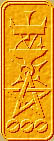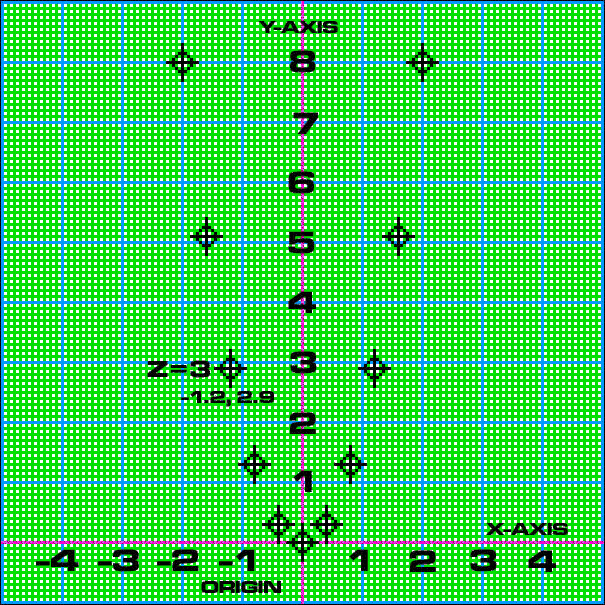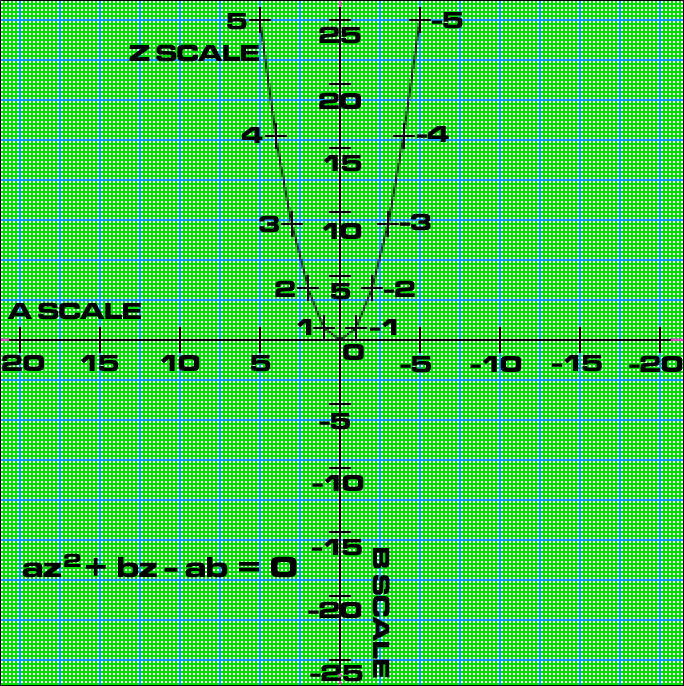Nomography Main > Determinants 01ρ=Σ+Ψ
``` <!-- RegisterNomoPage("determinant01.html") PrintNomoHeader("Reference", "Antique Instruments"); PrintNomoPageNum() //--> ```

# Creating Nomograms from Determinants

A Determinant is an operation on a square mathematical matrix. There are entire college courses on matrices and determinants (especially since they are powerful tools for 3-D computer graphics). But all you need to know are two things: [a] how to use a determinant to draw a nomogram and [b] how to turn an equation into a determinant.

Here is a skeleton of a three variable determinant:

```    |                |
| X(a)  Y(a)  1  |
|                |
| X(b)  Y(b)  1  | = 0
|                |
| X(c)  Y(c)  1  |
|                |
```

X( ) and Y( ) are functions, which in this case means its a fancy way of saying an equation with one variable. The variable is the one inside the parenthesis. X(a) and X(b) are totally different equations. For instance, X(a) might be a^2/(a-1) and X(b) might be -b.

Here is an actual real live determinant:

 -0.4 * a 0 1 -0.42 * z 0.32 * (z^2) 1 0 0.32 * b 1
= 0

It solves the quadratic equation az2 + bz - ab = 0 (as if you care) and has functional moduli that will fit it within 16 inches by 16 inches, a = +-20 and b = +- 25.

How do we draw it?

First we just look at the important part of the determinant:

 X coordequation Y coordequation a scale -0.4 * a 0 z scale -0.42 * z 0.32 * (z^2) b scale 0 0.32 * b

As you can see, the top row plots the a scale, the middle one the z scale and the bottom one the b scale. Each scale has two equations, one will calculate the X coordinate and the other the Y coordinate for a given value of the variable.

These two coordinates are used to plot a point for that value. Plotting is done with the Cartesian coordinate system.

Lets do an example to make things clear. We will plot the Z scale.

First make a table of X,Y values for the Z scale. You can do this by hand, but it is much easier to use a spreadsheet. Here is a sparse table for the Z scale:

 Z values X coord(-0.42 * z) Y coord(0.32 * (z^2)) -5 2.0 8.0 -4 1.6 5.1 -3 1.2 2.9 -2 0.8 1.3 -1 0.4 0.3 0 0.0 0.0 1 -0.4 0.3 2 -0.8 1.3 3 -1.2 2.9 4 -1.6 5.1 5 -2.0 8.0

Here is the Z scale plotted on Cartesian coordinates:The entire nomogram looks like this: